# Fe304Ca(CIO3)2BaCl2Cr2072-Cl2CO2H2SO3НС-Нз02C6H6Na2S203.S406²-Ca(CN)2

Question
1 views

Indicate the oxidation number of the underlined element in each of the following: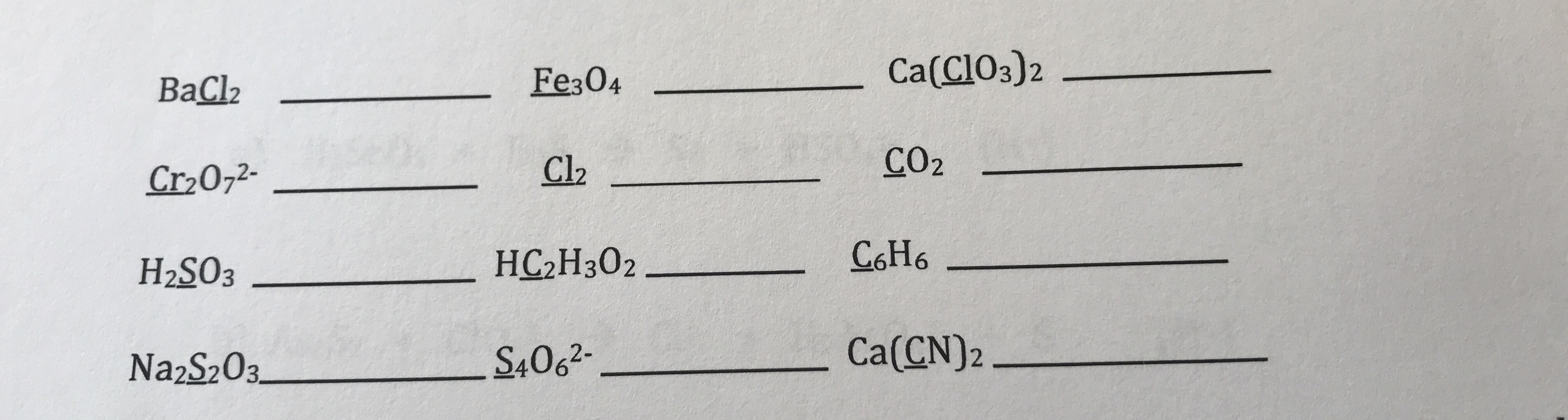help_outlineImage TranscriptioncloseFe304 Ca(CIO3)2 BaCl2 Cr2072- Cl2 CO2 H2SO3 НС-Нз02 C6H6 Na2S203. S406²- Ca(CN)2 fullscreen
check_circle

Step 1

Oxidation number of an element in the given compound represents the number of electrons lost (then oxidation state is positive) or gained(then oxidation state is negative) by that particular atom during the formtion of compound.

1)Free elements have oxidation state of zero. ex: Na ,K , Fe, S8 , H, Cl2 etc.

2) In a neutral compound, the sum of all the oxidation numbers is equal to zero.

3) In an ion, the sum of all the oxidation numbers is equal to the charge of the ion.

4) Usually the oxidation state of an atom is equal to its valency or group number.

ex: Group IA metals have +1 oxidation state as they lose their one valence electron easily to attain nearest inert gas configuration.

So, in NaCl, the oxidation state of sodium is +1.

Cl- is in VIIA group, it needs to gain one electron, so its oxidation number is -1.

5)Hydrogen always shows +1 oxidation state except in metal hydrides(NaH, CaH2 etc.) which is -1.

5) Except in peroxides (O22-) , superoxides (O2-) and ozone (O3) , oxygen mostly exhibits -2 oxidation state.

6) Fluorine always shows -1 oxidation state.

Step 2

Calculation of oxidation states of underlined elements in the given compounds:

Oxidation states of Cl in the given compounds:

Oxidation state of Cl in BaCl2:

Ba has +2 oxidation state as it belongs to IIA group.

Let the oxidation state of Cl be x.

Then,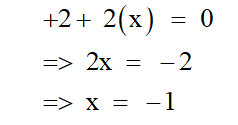Hence, the oxidation state of Cl in BaCl2 is -1.

Oxidation state of Cl in Cl2 is zero.

Cl2 is formed by mutual sharing of one electron by each Cl atom.

When the Cl-Cl bond breaks each Cl-atom takes back their electron and form the neutral Cl-atom again.

Hence, the oxidation state of Cl in Cl2 is zero.

Oxidation state of Cl in Ca(ClO3)2 :

Ca-atom has +2 oxidation state.

Let the oxidation number of Cl be x.

O-atom has oxidation number of -2.

Hence,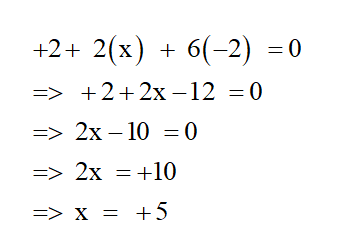Hence, the oxidation state of Cl in Ca(ClO3)2 is +5.

Step 3

Oxidation state of S in the given compounds:

H2SO3: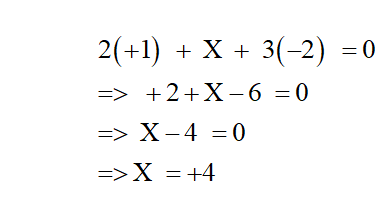Hence, the oxidation number of S in H2SO3 is +4.

Na2S2O3 :

The structure of Na2S2O3 is as shown below: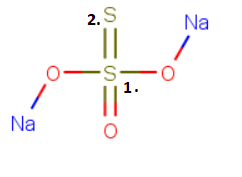Sulphur 1. has oxidation state +6

Sulfur 2. has oxidation state -2.

S4O62-:

Let the oxidation state of Sulfur be x.

O has -2 oxidation state.

Hence,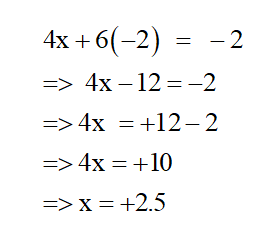...

### Want to see the full answer?

See Solution

#### Want to see this answer and more?

Solutions are written by subject experts who are available 24/7. Questions are typically answered within 1 hour.*

See Solution
*Response times may vary by subject and question.
Tagged in